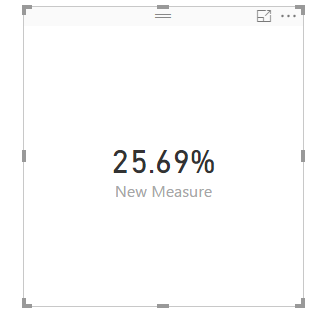cancel
Showing results for
Did you mean:Helper IV

## Calculating Measure

Hi,

I have this following situation where I want to calculate = Sum of (product sale by group)/Sum of Total product in Group

From this data, desired output is: = (599/2332) = 0.25

 Product Group Product sale by Group Total product in group P1 G1 78 122 P1 G2 24 48 P1 G3 18 76 P1 G4 49 160 P2 G1 39 123 P2 G2 6 41 P2 G3 19 57 P2 G4 55 152 P3 G1 39 139 P3 G2 7 31 P3 G3 5 71 P3 G4 17 132 P4 G1 18 122 P4 G2 30 47 P4 G3 13 61 P4 G4 9 115 P5 G1 8 122 P5 G2 16 49 P5 G3 2 115 P5 G4 40 123 P6 G1 14 95 P6 G2 26 66 P6 G3 9 121 P6 G4 58 144
1 ACCEPTED SOLUTIONMicrosoft

This will give you the result you say you want, regardless of what/where you filter.  Not sure if that is what you are after though

```New Measure = DIVIDE(
CALCULATE(
SUM(Table1[Product sale by Group]),
ALL('Table1')
)
,                    CALCULATE(
SUM(Table1[Total product in group]),
ALL('Table1')
)
)```

Proud to be a Datanaut!

3 REPLIES 3Microsoft

Hi @aktripathi2506,

As the @Phil_Seamark posted, create a measure using the formula and create a card to display the expected result as follows.Best Regards,
AngeliaMicrosoft

This will give you the result you say you want, regardless of what/where you filter.  Not sure if that is what you are after though

```New Measure = DIVIDE(
CALCULATE(
SUM(Table1[Product sale by Group]),
ALL('Table1')
)
,                    CALCULATE(
SUM(Table1[Total product in group]),
ALL('Table1')
)
)```

Proud to be a Datanaut!Responsive Resident

Hi

Not sure if I got you right. The formula of your measure would be:

```=DIVIDE(
SUM(product sale by group),
SUM(Total product in Group),
0)
```

Hope this helps

JJ